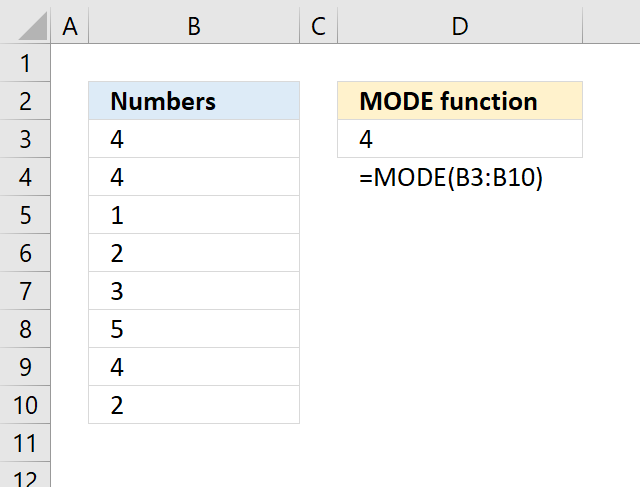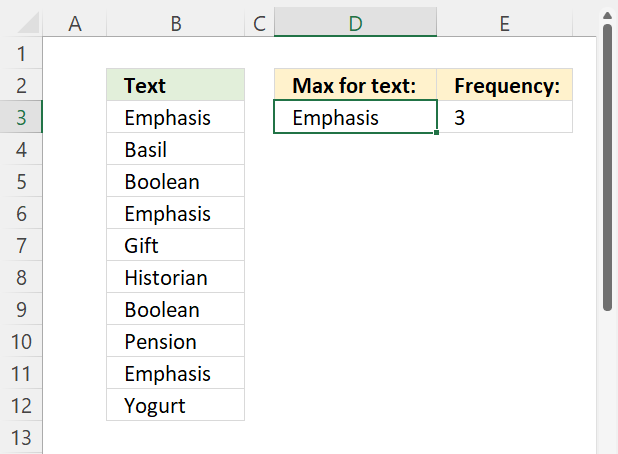Author: Oscar Cronquist Article last updated on May 05, 2022The MODE function calculates the most frequent number in a cell range.

Number 4 exists 3 times on picture above and number 2 exists only 2 times. Number 4 is the most frequent number in B3:B10.

### Excel Function Syntax

MODE(number1,[number2],...)

### Arguments

 number1 Required. A cell range you want to calculate the most frequent number. [number2] Optional. Up to 254 additional arguments.

### How to get the most common text valueFormula in cell D3:

=INDEX(B3:B12, MATCH(MODE(COUNTIF(B3:B12, "<"&B3:B12)), COUNTIF(B3:B12, "<"&B3:B12), 0))

### Explaining formula in cell D3

#### Step 1 - Create a number representing the position of each value in a sorted array

The COUNTIF function calculates the number of cells equal to a condition. In this case, the less than character compares all values and assigns a number to each value based on their position in a sorted array.

COUNTIF(rangecriteria)

COUNTIF(B3:B12, "<"&B3:B12)

becomes

COUNTIF({"Emphasis"; "Basil"; "Boolean"; "Emphasis"; "Gift"; "Historian"; "Boolean"; "Pension"; "Emphasis"; "Yogurt"},"<"&{"Emphasis"; "Basil"; "Boolean"; "Emphasis"; "Gift"; "Historian"; "Boolean"; "Pension"; "Emphasis"; "Yogurt"})

and returns

{3; 0; 1; 3; 6; 7; 1; 8; 3; 9}

Repeated values have identical numbers, we can use this to extract the most common number, see next step.

For example, "Boolean" is displayed twice in B3:B12, shown in the image above. The corresponding number in the array is 1, 1 is shown twice in the array.

#### Step 2 - Get the most repeated number

The MODE function returns the most common number in a cell range or array.

MODE(COUNTIF(B3:B12, "<"&B3:B12))

becomes

MODE({3; 0; 1; 3; 6; 7; 1; 8; 3; 9})

and returns 3. Number three is the most common number in the array.

#### Step 3 - Find the position of most repeated number

The MATCH function returns the relative position of an item in an array or cell reference that matches a specified value in a specific order.

MATCH(lookup_value, lookup_array, [match_type])

MATCH(MODE(COUNTIF(B3:B12, "<"&B3:B12)), COUNTIF(B3:B12, "<"&B3:B12), 0)

becomes

MATCH(3, COUNTIF(B3:B12, "<"&B3:B12), 0)

becomes

MATCH(3, {3; 0; 1; 3; 6; 7; 1; 8; 3; 9}, 0)

and returns 1. Three is the first value in the array.

#### Step 4 - Get value based on position

The INDEX function returns a value from a cell range, you specify which value based on a row and column number.

INDEX(array[row_num][column_num], [area_num])

INDEX(B3:B12, MATCH(MODE(COUNTIF(B3:B12, "<"&B3:B12)), COUNTIF(B3:B12, "<"&B3:B12), 0))

becomes

INDEX(B3:B12, 1)

becomes

INDEX({"Emphasis"; "Basil"; "Boolean"; "Emphasis"; "Gift"; "Historian"; "Boolean"; "Pension"; "Emphasis"; "Yogurt"}, 1)

and returns "Emphasis".

### How to get the most common text value - Excel 365Formula in cell D3:

=LET(z, B3:B12, y, COUNTIF(z, z), UNIQUE(FILTER(z, MAX(y)=y)))

### Explaining formula in cell D3

#### Step 1 - Count how many times each value is repeated in the array

The COUNTIF function calculates the number of cells equal to a condition.

COUNTIF(rangecriteria)

COUNTIF(B3:B12,B3:B12)

becomes

COUNTIF({"Emphasis"; "Basil"; "Boolean"; "Emphasis"; "Gift"; "Historian"; "Boolean"; "Pension"; "Emphasis"; "Yogurt"}, {"Emphasis"; "Basil"; "Boolean"; "Emphasis"; "Gift"; "Historian"; "Boolean"; "Pension"; "Emphasis"; "Yogurt"})

and returns {3; 1; 2; 3; 1; 1; 2; 1; 3; 1}.

#### Step 2 - Find the largest value

The MAX function returns the largest number in a cell range or array.

MAX(COUNTIF(B3:B12,B3:B12))

becomes

MAX({3; 1; 2; 3; 1; 1; 2; 1; 3; 1})

and returns 3.

#### Step 3 - Calculate the position of the largest value in array

The equal sign lets you compare value to value, it returns boolean values TRUE or FALSE.

MAX(COUNTIF(B3:B12,B3:B12))=COUNTIF(B3:B12,B3:B12)

becomes

3=COUNTIF(B3:B12, B3:B12)

becomes

3={3; 1; 2; 3; 1; 1; 2; 1; 3; 1}

and returns {TRUE; FALSE; FALSE; TRUE; FALSE; FALSE; FALSE; FALSE; TRUE; FALSE}.

#### Step 4 - Filter corresponding value

The FILTER function extract values/rows based on a condition or criteria.

FILTER(arrayinclude, [if_empty])

FILTER(B3:B12, MAX(COUNTIF(B3:B12,B3:B12))=COUNTIF(B3:B12,B3:B12))

becomes

FILTER(B3:B12, {TRUE; FALSE; FALSE; TRUE; FALSE; FALSE; FALSE; FALSE; TRUE; FALSE})

becomes

FILTER({"Emphasis"; "Basil"; "Boolean"; "Emphasis"; "Gift"; "Historian"; "Boolean"; "Pension"; "Emphasis"; "Yogurt"}, {TRUE; FALSE; FALSE; TRUE; FALSE; FALSE; FALSE; FALSE; TRUE; FALSE})

and returns {"Emphasis"; "Emphasis"; "Emphasis"}.

#### Step 5 - Extract unique distinct values

The UNIQUE function extracts both unique and unique distinct values and also compares columns to columns or rows to rows.

UNIQUE(array, [by_col], [exactly_once])

UNIQUE(FILTER(B3:B12, MAX(COUNTIF(B3:B12,B3:B12))=COUNTIF(B3:B12,B3:B12)))

becomes

UNIQUE({"Emphasis"; "Emphasis"; "Emphasis"})

and returns "Emphasis".

#### Step 6 - Shorten formula

The LET function lets you name intermediate calculation results which can shorten formulas considerably and improve performance.

LET(name1name_value1calculation_or_name2, [name_value2calculation_or_name3...])

The following formula can be shortened using the LET function, cell range B3:B12 is repeated many times in the formula.

Function COUNTIF(B3:B12,B3:B12) is also repeated in the formula, I have assigned z to B3:B12 and y to COUNTIF(B3:B12, B3:B12).

UNIQUE(FILTER(B3:B12, MAX(COUNTIF(B3:B12,B3:B12))=COUNTIF(B3:B12,B3:B12)))

becomes

LET(z, B3:B12, y, COUNTIF(z, z), UNIQUE(FILTER(z, MAX(y)=y)))